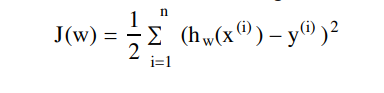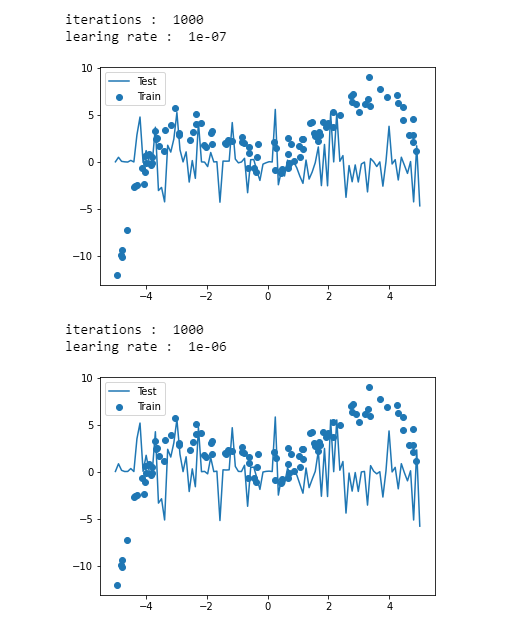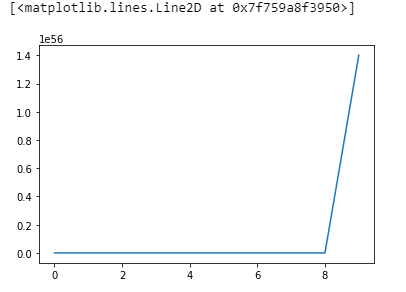top of page
Search

# Implementation of Multivariate Linear Regression to Solve the Polynomial Regression | Realcode4you

Implementation of multivariate linear regression to solve the polynomial regression problem:Below, you will follow steps to

1. Implement the cost function for multivarate linear regression

2. Compare vectorized code with for-loops

3. Implement the normal equations method to solve a multivariate linear regression problem

4. Implement gradient descent for multivariate linear regression

5. Experiment with feature normalization to improve the convergence of gradient descent

import all necessary packages

```% matplotlib inline
import numpy as np
import matplotlib.pyplot as plt
from IPython.display import display, clear_output

```

Helper functions

Run this code to set up the helper functions. The function feature_expansion accepts an vector of scalar x values and returns an n*5 data matrix by applying the feature expansionto each scalar value

```def feature_expansion(x, deg):
if x.ndim > 1:
raise ValueError('x should be a 1-dimensional array')
m = x.shape
x_powers = [x**k for k in range(0,deg+1)]
X = np.stack( x_powers, axis=1 )
return X

def plot_model(X_test, w):
'''
Note: uses globals x, y, x_test, which are assigned below
when the dataset is created. Don't overwrite these variables.
'''
y_test = np.dot(X_test, w)
plt.scatter(x, y)
plt.plot(x_test, y_test)
plt.legend(['Test', 'Train'])
```

List comprehensions

Read about Python list comprehensions. Explain what is happening in the following line of code

`x_powers = [x**k for k in range(0,deg+1)] `

Create a data set for polynomial regression

Read and run the code below. This generates data from a fourth-degree polynomial and then uses feature expansion to set up the problem of learning the polynomial as multivariate linear regression

```# Set random seed
np.random.seed(0)
# Create random set of m training x values between -5 and 5
m = 100
x = np.random.rand(m)*10 - 5
# Create evenly spaced test x values (for plotting)
x_test = np.linspace(-5, 5, 100)
m_test = len(x_test);
# Feature expansion for training and test x values
deg = 4
X = feature_expansion(x, deg)
X_test = feature_expansion(x_test, deg)
n = deg + 1 # total number of features including the '1' feature
# Define parameters (w) and generate y values
w = 0.1*np.array([1, 1, 10, 0.5, -0.5]);
y = np.dot(X, w) + np.random.randn(m) # polynomial plus noise
# Plot the training data
plt.scatter(x, y)
plt.title('Training Data')
plt.xlabel('x')
plt.ylabel('y')
plt.show()

```

Output:```#look at the feature expansion for a single training example
print(x)  #original data
print(X)  #data with feature expansion ```

output:

0.48813503927324753

[1. 0.48813504 0.23827582 0.11631078 0.05677536]

Implement the cost function

Follow the instructions to implement the following cost function for multivariate linear regression:Cost function with loops

First, implement the cost function using a for-loops: cost_function_loops .

```def cost_function_loops(X, y, w):
'''
Compute the cost function for a particular data set and
hypothesis (parameter vector)

Inputs:
X m x n data matrix
y training output (length m vector)
w parameters (length n vector)
Output:
cost the value of the cost function (scalar)
'''
# TODO: write correct code to implement the cost function given above
# Use for-loops for this function
cost = 0
return cost
```

Implementation of above function:

```def cost_function_loops(X, y, w):
'''
Compute the cost function for a particular data set and
hypothesis (parameter vector)

Inputs:
X m x n data matrix
y training output (length m vector)
w parameters (length n vector)
Output:
cost the value of the cost function (scalar)
'''
# TODO: write correct code to implement the cost function given above
# Use for-loops for this function
Hypothesis = np.dot(X,w)
Error = y - Hypothesis
#Matrix method for calculating Cost
Cost = np.dot(Error.T,Error)/(2*len(Error))

#The loop method for calculatin Cost
for i in range(0,len(Error)):
Cost = Cost + Error[i]*Error[i]
Cost = Cost/(2*len(Error))
return Cost

```

Vectorized cost function

```def cost_function_vec(X, y, w):
'''
No for-loops allowed!

Compute the cost function for a particular data set and
hypothesis (parameter vector)

Inputs:
X m x n data matrix
y training output (length m vector)
w parameters (length n vector)
Output:
cost the value of the cost function (scalar)
'''

# TODO: write correct code to implement the cost function given above
# You CANNOT use for-loops here!
m = y.size
error = np.dot(X, w.T) - y
cost = 1/(2*m) * np.dot(error.T, error)
return cost
```

Test the cost function

```
np.random.seed(1)
w_random = np.random.rand(n)
w_zeros = np.zeros(n)
w_ones = np.ones(n)
print("cost_functioon_loops")
print("=="*10)
print( "Cost (random): %.2f" % cost_function_loops(X, y, w_random)) # prints 54523.64
print( "Cost (zeros): %.2f" % cost_function_loops(X, y, w_zeros)) # prints 845.65
print( "Cost (ones): %.2f" % cost_function_loops(X, y, w_ones)) # prints 2524681.08
print()
print("cost_function_vec")
print("=="*10)
print( "Cost (random): %.2f" % cost_function_vec(X, y, w_random)) # prints 54523.64
print( "Cost (zeros): %.2f" % cost_function_vec(X, y, w_zeros)) # prints 845.65
print( "Cost (ones): %.2f" % cost_function_vec(X, y, w_ones)) # prints 2524681.08
print()

#Note: The for-loop and vectorized cost function implementations should return the EXACT
# same results.

```

output:

cost_functioon_loops ==================== Cost (random): 547.96 Cost (zeros): 8.50 Cost (ones): 25373.04 cost_function_vec ==================== Cost (random): 545.24 Cost (zeros): 8.46 Cost (ones): 25246.81

Test the cost function

```
np.random.seed(1)
w_random = np.random.rand(n)
##################
# TODO: implement your code here #
import timeit
print('cost_function_loops')
start = timeit.default_timer()
cost_function_loops(X, y, w_random)
stop = timeit.default_timer()
print('Time: ', stop - start)

print('cost_function_vec')
start = timeit.default_timer()
cost_function_vec(X, y, w_random)
stop = timeit.default_timer()
print('Time: ', stop - start)
##################

```

output:

```cost_function_loops
Time:  0.00016652100021019578
cost_function_vec
Time:  0.0025312670004495885```
`cost_function = cost_function_vec`
```#make sure it works
cost_function(X, y, w_random)```

output:

`545.2364276966484`

Test the cost function

```def normal_equations(X, y):
X = np.array(X)
m,n = X.shape

#using normal equation
theta = np.dot(np.dot(np.linalg.inv(np.dot(X.T, X)),X.T), y)
return theta```

Use normal equations to fit the model

```
w_normal_equations = normal_equations(X, y)
plot_model(X_test, w_normal_equations)
print ("Cost function: %.2f" % cost_function(X, y, w_normal_equations))

```

Cost function: 0.49```Implement second training algorithm: (vectorized) gradient descent

def gradient_descent( X, y, alpha, iters, w=None ):
m,n = X.shape
​
if w is None:
w = np.zeros(n)
​
# For recording cost function value during gradient descent
J_history = np.zeros(iters)
for i in range(iters):
predictions = X.dot(w)
#print('predictions= ', predictions[:5])
errors = np.subtract(predictions, y)
#print('errors= ', errors[:5])
sum_delta = (alpha / m) * X.transpose().dot(errors);
#print('sum_delta= ', sum_delta[:5])
w = w - sum_delta;
​
J_history[i] = cost_function(X, y, w)
​
return w, J_history```

Use gradient descent to train the model

```w,history =gradient_descent( X, y, 0.0001,100)
plot_model(X,w)```

output:```plt.plot(history)
plt.xlabel('iterations')
plt.ylabel('cost')
plt.show()
print('final value of cost : ',history[-1])```

output:```for lr in [0.001,0.000001,0.0000001,0.000001]:
print('iterations : ',1000)
print('learing rate : ',lr)
plot_model(X,w)
plt.show()```

output:

iterations : 1000 learing rate : 0.001 /usr/local/lib/python3.7/dist-packages/ipykernel_launcher.py:16: RuntimeWarning: invalid value encountered in subtract app.launch_new_instance()it takes 100 iterations and learning rate 1e-6 to get it close to normal equation

`plot_model(X,w)`

output:`plt.plot(history)`

output: